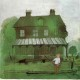## A Problem on Planar Percolation

Conjecture (Gady Kozma):  Prove that the critical probability for planar percolation on a Cayley graph of the group$Z^2$ is always an algebraic number.

Gady  mentioned this conjecture in his talk here about percolation on infinite Cayley graphs.  (Update April 30: Today Gady mentioned to me an even bolder question: to show that for every group$\Gamma$, either all critical probabilities of its Cayley graphs are algebraic, or none is!!;  I recall a similarly bold conjecture regarding the property of being an expander which turned out to be false but was fruitful nevertheless.)

Many problems on percolation beyond$Z^d$ were posed by Benjamini and Schramm, (See here (the paper) and here (update web page  1999)). In the talk the beautiful proof by Itai, Russ, Yuval, and Oded of the “dying percolation property” for nonamenable groups was outlined.  And the Burton-Keane beautiful result on the unique infinite connected component for the amenable case was mentioned.

Brief explanations: You have an infinite graph$G$. Consider a random subgraph$H$ where every edge of$G$ is taken with probability$p$. (The edges taken are called  open, and the edges not taken are called closed.) We ask:

What is the probability$\Theta(p)$  that$H$ has an infinite connected component? (Kolmogorov’s 0-1 law asserts that it is zero or one.)

Now,$\Theta(p)$ is also monotone and the critical probability is the supremum of the set of all$p$ so that$\Theta(p)$ is 0.

The dying percolation property asserts that$\Theta(p_c)=0$.  It is known to hold for the standard planar percolation and is a notorious open problem for standard percolation in three dimensions.

Under very very general conditions the number of infinite components is, with probability 1, either zero, one or infinity. There is a beautiful proof of Burton and Keane that for percolation on Cayley graphs of amenable groups, above the critical percolation$H$ has, with probability one, a unique infinite component. It is conjectured that for the nonamenable case there is always an interval above the critical probablity where$H$ has infinitely many components!This entry was posted in Open problems, Probability and tagged , . Bookmark the permalink.

### 1 Response to A Problem on Planar Percolation

1.Gábor Pete says: